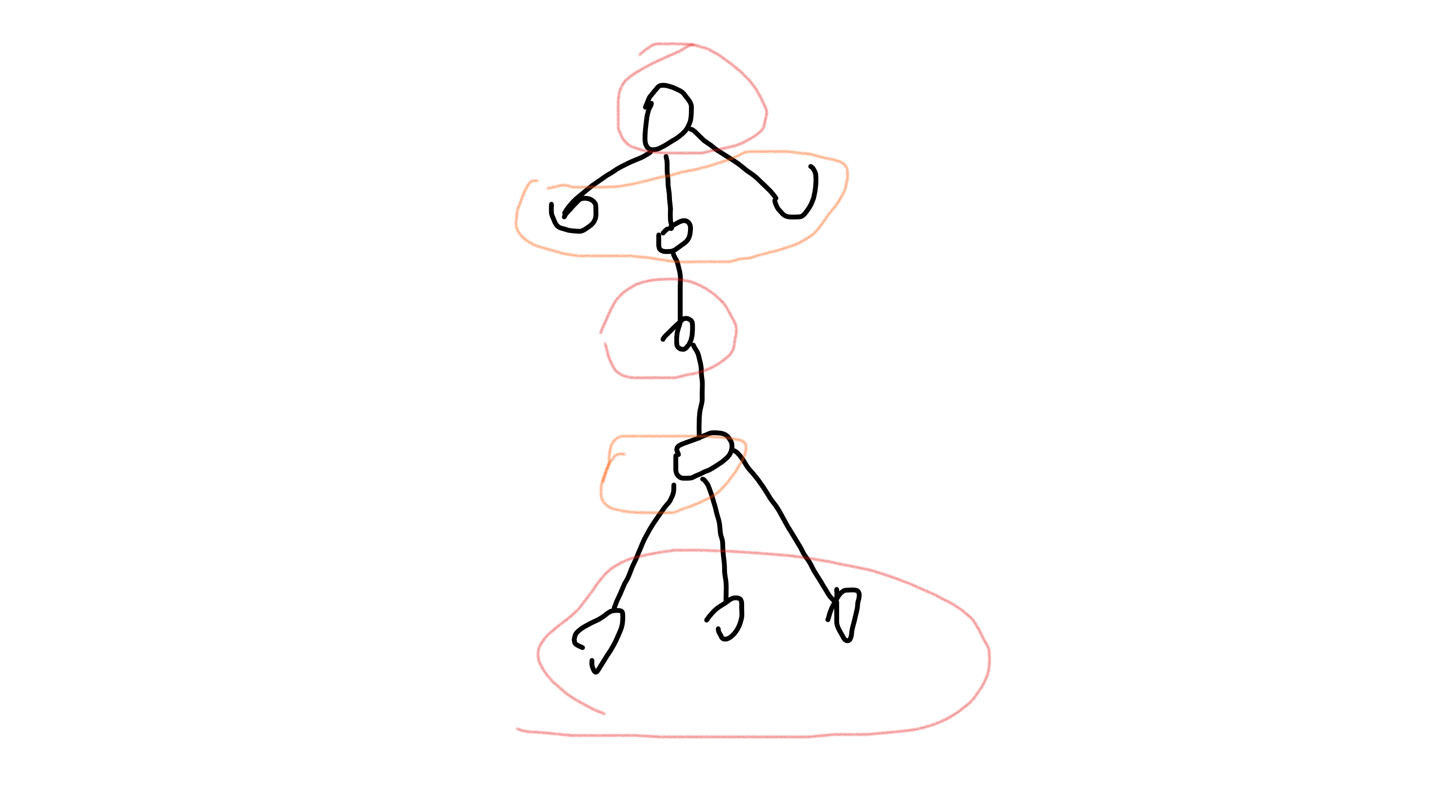# UVA1220 - Party at Hali-Bula

• $f[u] = \sum\limits_{v \in son[u]} \max(f[v], f[v])$
• $f[u] = \sum\limits_{v \in son[u]} f[v]$

• 如果当前节点 $u$ 更新状态选中的来自 $v$ 的某个状态存在多种，那么 $u$ 的这个状态也存在多种。
• 如果 $u$ 节点的某个孩子 $v$ 满足 $f[v] == f[v]$ ，那么 $f[u]$ 存在多种。

# 分析

[L, R]表示闭区间，(L, R)表示开区间！）

# LJOJ1244 - 埃及分数

• 迭代加深
本题中，我们限定DFS搜索的层数，如果超过这个层数，就进行剪枝。

• 启发式搜索
我们设立一个估价函数，考虑最优情况下的步数。
本题中我们按照分母从小到大的顺序来DFS埃及分母，所以之后遇到的值就必定大于当前取到的值。
我们可以通过这个特性来写估价函数，即(a / b) / (1 / u)（其中a / b表示当前的值，u表示剩下能够取到的最小分母）
做其他题目时一定要注意估价函数所估计的值之多劣于实际情况，而不能比实际情况更优

# 洛谷2996 - 拜访奶牛• 如果当前节点不选，那么孩子节点选或不选均可
$f[u] += max(f[G[u][i]], f[G[u][i]])$

• 如果当前节点选中，那么孩子节点只能不选
$f[u] += f[G[u][i]]$

# 洛谷2921 - 在农场万圣节

• 环内节点的答案 = 环的长度；
• 环外节点的答案 = 环的长度 + 当前节点到环的距离。